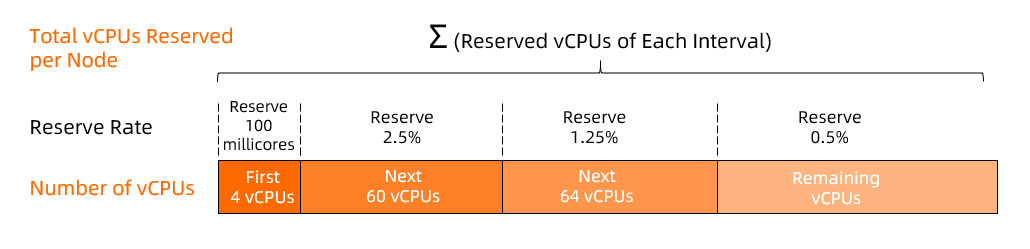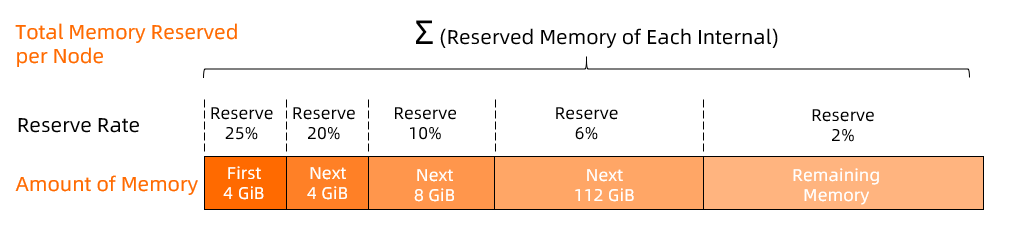Container Service for Kubernetes (ACK) requires a specific amount of computing resources to run components such as kubelet, kube-proxy, Terway, and the container runtime. Consequently, the amount of allocatable resources in a cluster is less than the total amount of resources in the cluster. This topic describes the resource reservation policy of ACK and the considerations of the policy. This helps you set proper resource requests and limits for pods.

## Check the allocatable resources of a node.

Run the following command to query information about the total resources and allocatable resources of a node:

``kubectl describe node [NODE_NAME] | grep Allocatable -B 7 -A 6``
Expected output:
``````Capacity:
cpu:                4                 # The total number of vCPUs of the node.
ephemeral-storage:  123722704Ki       # The total amount of ephemeral storage of the node. Unit: KiB.
hugepages-1Gi:      0
hugepages-2Mi:      0
memory:             7925980Ki         # The total amount of memory of the node. Unit: KiB.
pods:               64
Allocatable:
cpu:                3900m             # The number of vCPUs that can be allocated from the node.
ephemeral-storage:  114022843818      # The amount of ephemeral storage that can be allocated from the node. Unit: KiB.
hugepages-1Gi:      0
hugepages-2Mi:      0
memory:             5824732Ki         # The amount of memory that can be allocated from the node. Unit: KiB.
pods:               64``````

## Calculate the amount of allocatable resources

The amount of allocatable resources = The total amount of resources - The amount of reserved resources - The eviction threshold

Where:

• The total amount of resources can be obtained from the `Capacity` field of the preceding output.

## Resource reservation policy

The following factors are taken into consideration when ACK reserves the resources of nodes:

• In most cases, Elastic Compute Service (ECS) nodes of high specifications run a large number of pods. To ensure node performance, ACK reserves more resources for Kubernetes components.
• Windows nodes require additional resources to run the Windows operating system and components related to Windows Server. In this case, ACK reserves more resources for a Windows node than ACK does for a Linux node. For more information, see Create a Windows node pool.

ACK reserves vCPUs and memory resources at different rates as the amount of CPU and memory resources increases.

• The reserved vCPUs are calculated as described in the following figure.For example, the number of reserved vCPUs for a node with 32 vCPUs is calculated based on the following formula:

100 + (32,000 - 4,000) × 2.5% = 800 millicores

• The reserved memory is calculated as described in the following figure.For example, the amount of reserved memory for a node with 256 GiB of memory is calculated based on the following formula:

4 × 25% + (8 - 4) × 20% + (16 - 8) × 10% + (128 - 16) × 6% + (256 - 128) × 2% = 11.88 GiB

## Considerations

• The resource reservation policy applies only to Kubernetes 1.16.9 and later
• For more information about how to modify the amount of reserved resources, see Customize the kubelet configurations of a node pool.
• The resource reservation policy is automatically applied after a node is created. Manual configuration is not required.
• Take note of the following items for existing nodes:
• To ensure node stability, the resource reservation policy is not applied to existing nodes that have not been managed by performing node pool O&M operations, such as customizing node pool configurations and updating node pools.
• The resource reservation policy is automatically applied to existing nodes after you update the clusters to which the nodes belong, update the node pools to which the nodes belong, or customize the kubelet parameters for the node pools to which the nodes belong.
• Changing the amount of reserved resources may reduce the amount of allocatable resources of existing nodes. If the resource usage of an existing node is high, workloads on the node may be evicted.
• If you want to apply the resource reservation policy to an existing node, remove the node from the cluster and then add the node to the cluster again. This way, the resource reservation policy is automatically applied to the node after the node is added. For more information about how to remove a node from a cluster, see Remove a node.